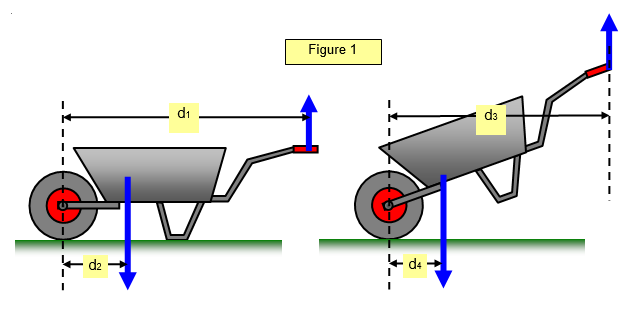# The wheelbarrowYou should be able to prove that as you tip the wheelbarrow the size of the force required in the handles does not change. (This assumes that the load does not slip forward). The distances between the load and pivot and effort and pivot are both affected in the same way.

Example problem
(a) A wheelbarrow and its load has a mass of 25 kg and a common centre of gravity 0.5 m from the vertical line through the axle. If the handles are 1.25 m from the pivot calculate the force needed to keep the wheelbarrow horizontal. (Take g = 9.8 ms-2)

Force = [25x9.8x0.5]/1.25 = 98 N

(b) the wheelbarrow is now titled so that it makes an angle of 35o with the horizontal. Calculate the vertical force needed at the handle to keep it in this position.

Force = [25x9.8xcos35]/1.25cos35 = 98N the same as before.

If you actually try this you will find that it seems to get harder to tip a wheelbarrow. This is because you cannot keep your arms pulling in a vertical line above the handles as the barrow is tilted unless you are exceptionally tall.

A VERSION IN WORD IS AVAILABLE ON THE SCHOOLPHYSICS USB